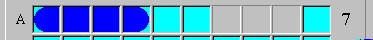Solver Rule: The rest of the row/column consists of ship segments

If all water squares in a row/column have been found, the rest of the row/column consists solely of ship segments.

ExampleIn the example above, row A has a row tally of seven (that's the number to the right of the row). This means there are exactly seven ship segments hidden in row A. In other words, there are exactly three water squares to be found in row A. Since the three water squares have been found at (A,5), (A,6) and (A,10), the remaining unknown squares (A,7)-(A,9) must be ship segments.